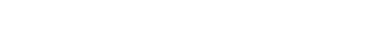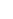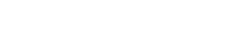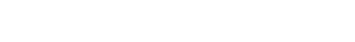# Minimum Thickness of Two Way Slab as per ACI 318-11 for Deflection ControlReading time: 1 minute

Minimum thickness for two way slab construction designed as per methods provided by ACI 318-11 Code namely, direct design method and equivalent frame method. The minimum thickness of slab is specified by ACI 318-11, section 9.5.3. Deflection of two way slab could be calculated by using available methods to make sure that the deflection will not be problematic and will not exceed serviceability limitations.

## Minimum Thickness of Two Way Slab as per ACI 318-11 for Deflection Control

The deflection of two way slab is based on a number of parameters for example the flexural stiffness of the slab which is the function of slab thickness. The flexural stiffness of the slab is increased with increasing the thickness of the slab; as a result the deflection of the two-way slab is declined. In order to prevent excessive deflections and avoid calculation of two way slab deflection which is a complicated procedure, ACI 318-11 restricts the minimum thickness of two way slab by applying three empirical limitations. It will not be necessary to carry out deflection computation if these limitations are met. Finally, slab thicknesses smaller than those obtained from empirical limitations can be employed if deflection calculation is within specified limits which are determined by Code. ACI Code limitations for minimum thickness of two way slab are discussed in the following sections. 1. For afm larger than 0.2 but not larger than 2, the slab thickness must not be less than the values of equation-1:Where: h: Minimum slab thickness ln: clear span measured in long direction face to face of column or face to face of beam for slabs with beams: Ratio of clear span in longer direction to clear span in shorter direction afm: Average value of (af) for all beams on the sides of a panel af: is the ratio of flexural stiffness of the beam section to the flexural stiffness of the slab bounded laterally by centerline of the panel on each side of the beam. Computation of is according to the following equation:Where: Ecb and Ecs: concrete modulus of elasticity of beam and slab respectively which is usually the same Ib and Is: Moment of inertia of beam and slab respectively. Figure-1 & Figure-2 illustrate how to find moment of inertia for edge beam, internal beam, internal and edge slabs respectively:Figure-1: Portion of slabs to be included for moment of inertia calculation, edge beam (left side) & internal beam (right side)Figure-2: Dimensions of internal and external slab for moment of inertia calculations

2. For (afm) larger than 2, the thickness of the slab must not be less than the value of equation-2:3. For (afm) equal or smaller than 0.2, minimum two-way slab thickness is provided by Table-1.

Table-1: Minimum thickness of slabs without interior beamsMoreover, the minimum thickness of any two way slab without interior beams should not be less than the following:
• For slabs without drop panel 125 mm.
• For slabs with drop panel 100 mm.
Furthermore, both (afm) and (af) will be zero when beams are not employed as in the case of flat plates. Lastly, ACI Code equations applied to calculate slab thickness take the panel shape, the influence of span length, the flexural stiffness of beams, and the yield stress of steel reinforcement into consideration. Equation-3 will control the slab thickness if substantially stiff beam is used since equation-1 gives smaller thickness. If no beams are used for example in the case of flat plates and flat slabs, the minimum thickness of the slab could be taken from table-1. Read More: Two Way Slab Design by Direct Design Method as per ACI 318-11